# Recognise Correlation and Causation

In this worksheet, student practise recognising correlation and causation in scatter graphs.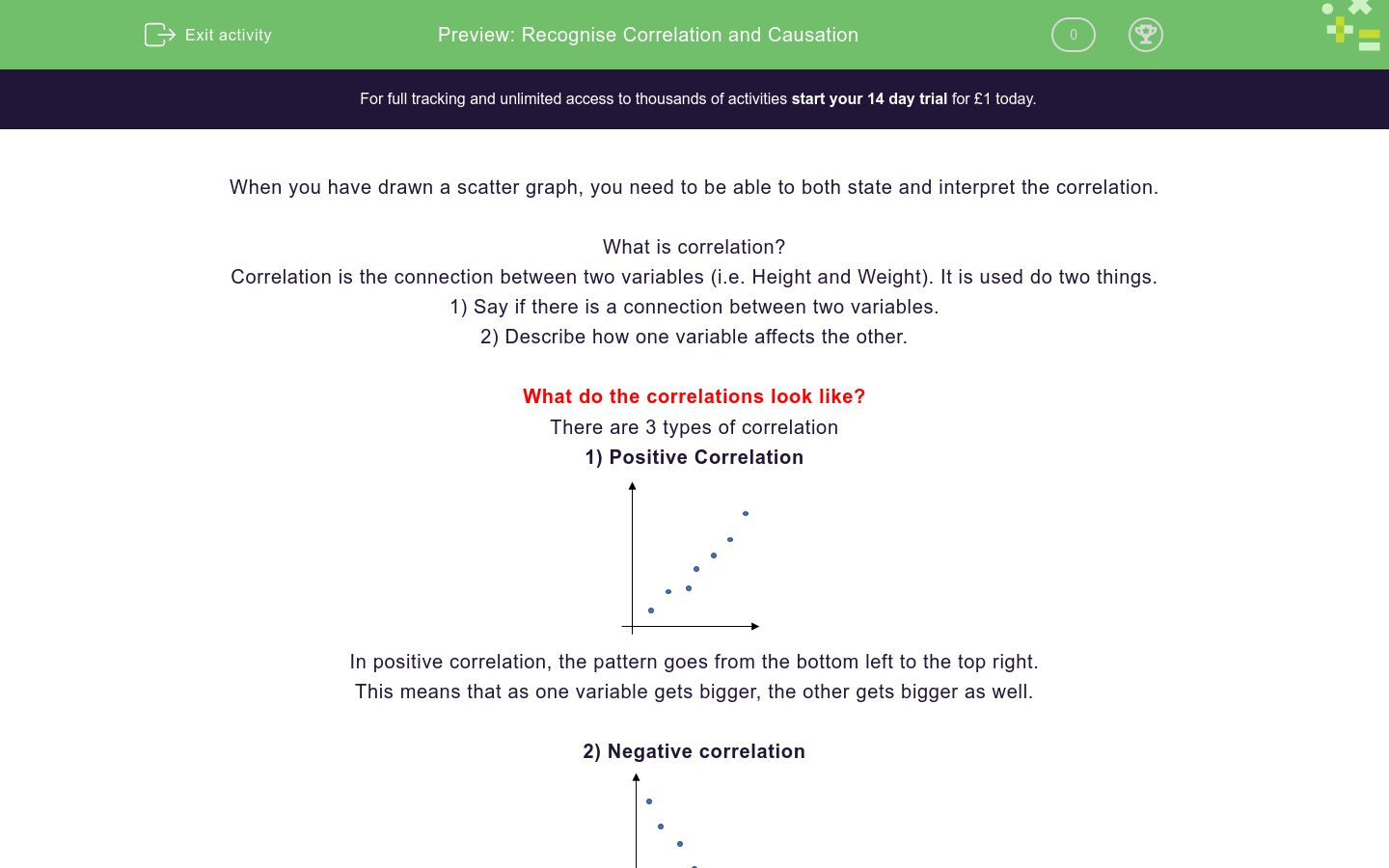Key stage:  KS 4

GCSE Subjects:   Maths

GCSE Boards:   AQA, Eduqas, Pearson Edexcel, OCR

Curriculum topic:   Statistics

Curriculum subtopic:   Statistics, Analysing Data

Difficulty level:### QUESTION 1 of 10

When you have drawn a scatter graph, you need to be able to both state and interpret the correlation.

What is correlation?

Correlation is the connection between two variables (i.e. Height and Weight). It is used do two things.

1) Say if there is a connection between two variables.

2) Describe how one variable affects the other.

What do the correlations look like?

There are 3 types of correlation

1) Positive Correlation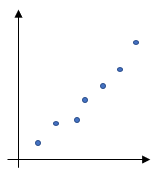In positive correlation, the pattern goes from the bottom left to the top right.

This means that as one variable gets bigger, the other gets bigger as well.

2) Negative correlation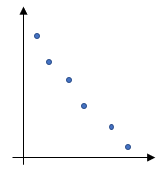In negative correlation, the pattern goes from the top left to the bottom right.

This means that as one variable gets bigger, the other gets smaller.

3) No correlation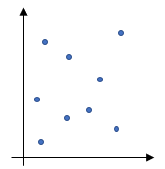When the points are scattered all over the graph, there isn't a pattern that links the two so we say there is no correlation

What is causation?

Causation is a bit trickier to get your head round, sometimes you have to use common sense.

If you plotted a graph of height against weight, you would get a positive correlation. This is also causation because the two are linked, it makes sense to say that the taller you are, the more you weigh.

If however, you plotted the number of pirates in the world against the average global temperature, you would get a negative correlation. Does this mean that the more pirates there are in the world, the lower the temperature? Does it make sense that the two are linked?

This is an example of the fact that correlation does not imply causation.

Just because you have two thinks that appear to be linked, it doesn't always mean that one causes the other.

The three types of correlation are ...

Match the correlation with the graph

## Column B

Positive

');" onmouseout="tooltip.hide();">

[Image_Link]/userfiles/image/Active%20Imag...

Negative

');" onmouseout="tooltip.hide();">

[Image_Link]/userfiles/image/Active%20Imag...

None

');" onmouseout="tooltip.hide();">

[Image_Link]/userfiles/image/Active%20Imag...

Is this correlation...Positive

Negative

none

Complete the sentence...

Positive

Negative

none

For each event, select if the two things cause each other or not.

Does this scatter graph show that A increases as B increases?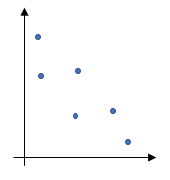Yes

No

Which of these two scatter graphs shows a stronger correlation?Which of these two scatter graphs shows a weaker correlation?For each situation, select if you think it will be a positive correlation, a negative correlation or have no correlation.

Does this scatter diagram show...Correlation

Causation

• Question 1

The three types of correlation are ...

EDDIE SAYS
These are just ones that you need to learn, positive, negative and no correlation
• Question 2

Match the correlation with the graph

## Column B

Positive

');" onmouseout="tooltip.hide();">

[Image_Link]/userfiles/im...

Negative

');" onmouseout="tooltip.hide();">

[Image_Link]/userfiles/im...

None

');" onmouseout="tooltip.hide();">

[Image_Link]/userfiles/im...

-->
EDDIE SAYS
Remember: Positive goes up Negative goes down None is scattered all over the place
• Question 3

Is this correlation...Positive
EDDIE SAYS
While this does look a bot more scattered, we could still draw a line through the points from the bottom left to top right. We call this a weak positive
• Question 4

Complete the sentence...

EDDIE SAYS
In an exam, you will probably only be asked if one variable causes another. The key word in these questions is causation
• Question 5

For each event, select if the two things cause each other or not.

EDDIE SAYS
The ones you need to consider here are the last two. Does it makes sense that the older you are, the more you earn? Does hair colour change as you get older?
• Question 6

Does this scatter graph show that A increases as B increases?No
EDDIE SAYS
A increasing as B increases would be a positive correlation, this is a ...
• Question 7

Which of these two scatter graphs shows a stronger correlation?EDDIE SAYS
These are both positive correlations, when we want the strongest correlation, we need to say which ones are closer to being in a straight line.
• Question 8

Which of these two scatter graphs shows a weaker correlation?EDDIE SAYS
When we are looking for a weaker correlation, we have to decide which is the least like a straight line. If we have a negative correlation and a no correlation, which one is least like a straight line?
• Question 9

For each situation, select if you think it will be a positive correlation, a negative correlation or have no correlation.

EDDIE SAYS
Remember: Positive correlation means as one gets bigger so does the other Negative correlation means as one gets bigger the other gets smaller No correlation means that they aren\'t linked.
• Question 10

Does this scatter diagram show...Correlation
Causation
EDDIE SAYS
They\'re both there. They are correlated because the scatter graph shows positive correlation We can also say that increasing height will cause an increase in weight,
---- OR ----

Sign up for a £1 trial so you can track and measure your child's progress on this activity.

### What is EdPlace?

We're your National Curriculum aligned online education content provider helping each child succeed in English, maths and science from year 1 to GCSE. With an EdPlace account you’ll be able to track and measure progress, helping each child achieve their best. We build confidence and attainment by personalising each child’s learning at a level that suits them.

Get started